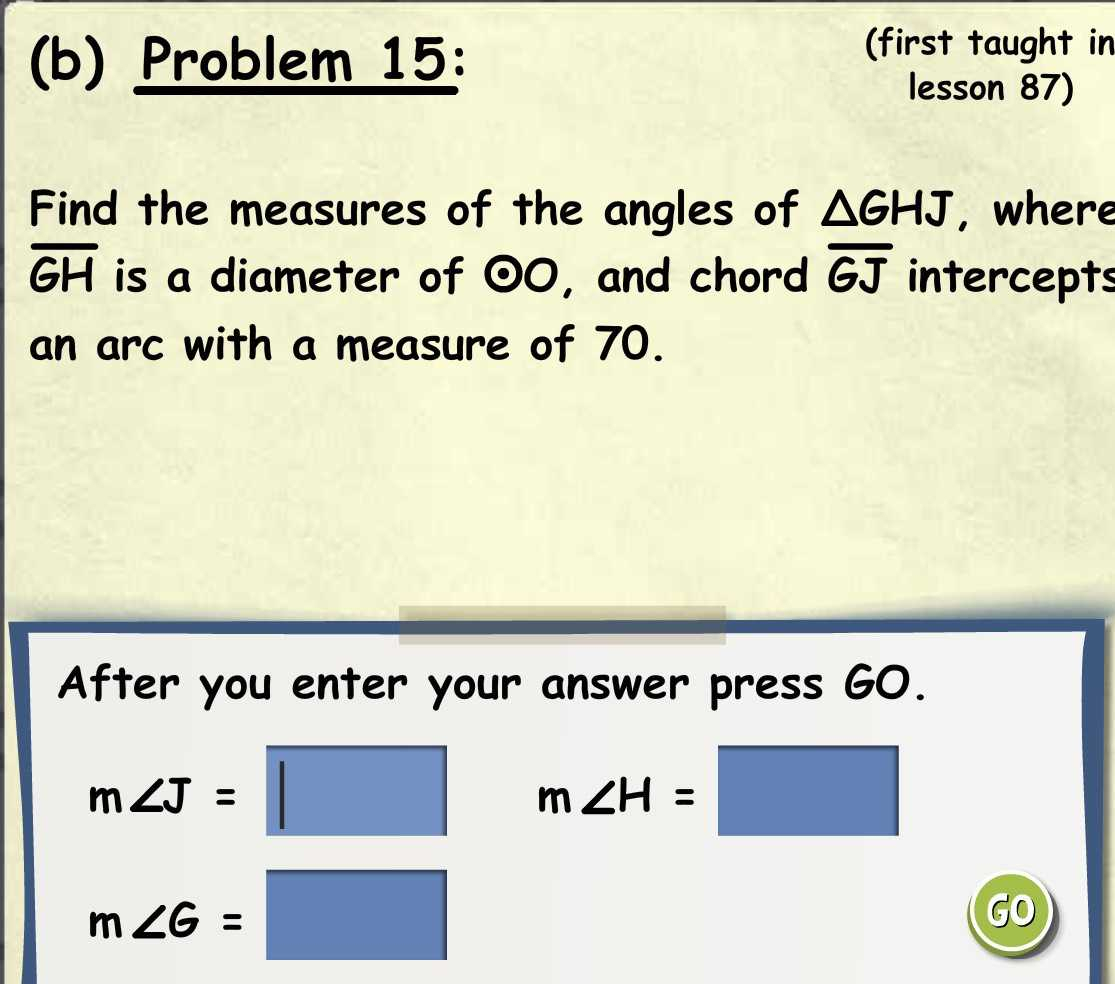### Still have math questions?

Trigonometry
QuestionFind the measures of the angles of $$\triangle G H J$$ , where  $$\overline { G H }$$ is a diameter of $$O 0$$ , and chord $$\overline { G J }$$ intercepts an arc with a measure of $$70$$ .

$$m \angle J =$$

$$m \angle H =$$

$$m \angle G =$$

$$\angle J= 90°$$
$$\angle H= 35°$$
$$\angle G= 55°$$